# Sets in Julia

Set in Julia is a collection of elements just like other collections like arrays, dictionaries, etc. Sets are different from arrays because sets are unordered collections of unique elements whereas the order of elements is strictly remembered in Arrays. Also, Arrays and dictionaries may contain duplicate values but Sets can’t. Each element in a set must be unique. Sets are mutable data types, which means its values can be altered, deleted, overwritten, etc.

Sets can contain elements of any data type. Just like other collections, it is not mandatory in Julia to define the data type of a set before the declaration of the elements. Julia automatically analyzes the elements and defines the Set’s data type. Julia doesn’t allow to access elements of a set individually because it is an unordered collection and hence, there is no fixed index of any element.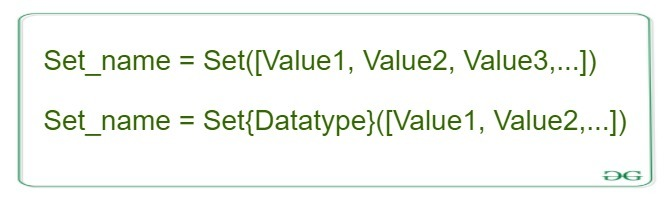#### Creating a Set

A set in Julia can be created with a pre-defined keyword `Set()`. This keyword accepts elements placed within square brackets([]) and creates a set by defining its data type based on the data type of the elements.
Syntax:

```Set_name = Set([Value1, Value2, Value3, ....])
```

 `# Julia program to illustrate ` `# the use of Sets ` ` `  `# Creating an empty set ` `Set1 ``=` `Set``() ` `println(``"Empty Set: "``, Set1) ` ` `  `# Creating a set with Integer values ` `Set2 ``=` `Set``([``1``, ``2``, ``3``, ``4``, ``5``, ``2``, ``4``, ``6``]) ` `println(Set2) ` ` `  `# Creating a set with mixed datatypes ` `Set3 ``=` `Set``([``1``, ``2``, ``3``, ``"Hello"``, ``"Geeks"``]) ` `println(Set3) `

Output: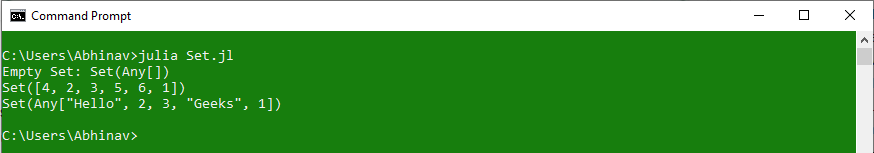#### Accessing elements in a Set

Set items cannot be accessed by referring to an index value, since sets are unordered the items have no fixed index. But you can loop through the set items using a for loop, or ask if a specified value is present in a set, by using the `in()` keyword.

 `# Julia program to demonstrate  ` `# Accessing of elements in a set  ` `   `  `# Creating a set  ` `Set1 ``=` `Set``([``"Geeks"``, ``"For"``, ``"Geeks"``])  ` `println(``"\nInitial set:"``)  ` `println(Set1)  ` `   `  `# Accessing element using  ` `# for loop  ` `println(``"\nElements of set: "``)  ` `for` `i ``in` `Set1  ` `    ``println(i)  ` `end ` `   `  `# Checking the element  ` `# using in keyword  ` `println(``in``(``"For"``, Set1)) `

Output: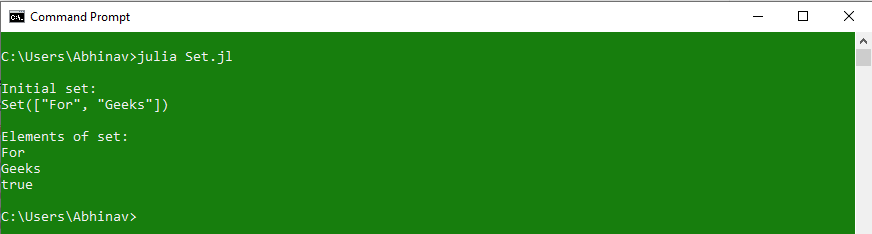#### Methods for Sets

Sets are mutable type collections in Julia, hence, their values can be modified with the use of certain pre-defined keywords. Julia allows adding new elements in a set with the use of `push!` command. Adding elements to a set is quite similar to adding elements in an array, the only difference is, unlike arrays, elements in set can’t be added on a specific index because sets are unordered.
Syntax:

```push!(Set_name, Value)
```

 `# Julia program to demonstrate  ` `# Accessing of elements in a set  ` `    `  `# Creating a set  ` `Set1 ``=` `Set``([``"Hello"``, ``"Geeks"``, ``10``])  ` `println(``"\nInitial set:"``)  ` `println(Set1)  ` `  `  `# Adding an element to the set ` `Set1 ``=` `push !(Set1, ``"Welcome"``) ` `println(``"\nSet after adding one element:\n"``, Set1) ` `  `  `# Adding a range of elements ` `# using for loop ` `for` `i ``in` `1``:``5` `    ``push !(Set1, i) ` `end ` `println(``"\nSet after adding range of elements:\n"``, Set1) `

Output: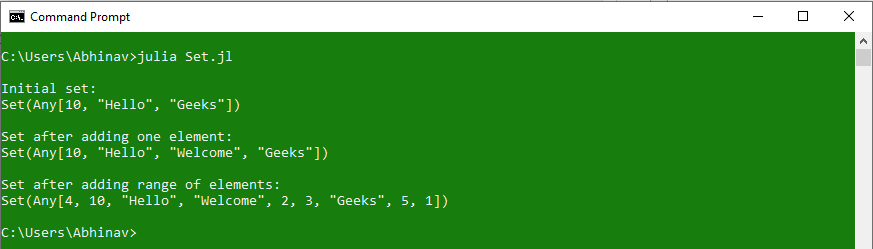#### Union of Sets

Julia allows merging elements of two sets with the use of `union` keyword. Union operation takes both the sets as an argument and gives the result as another set having elements of both the sets. The actual values of both sets do not change unless the return value is stored in any of the two sets. There’s another variant of `union` function which is `union!`. Both the keywords perform similar operations, but the only difference is that the `union!` function will overwrite the existing set with the new merged set.

Syntax:

```union(Set1, Set2)
or
union!(Set1, Set2)
```

Example 1: Performing union operation

 `# Julia program to demonstrate  ` `# Accessing of elements in a set  ` `   `  `# Creating a set  ` `Set1 ``=` `Set``([``"Hello"``, ``"Geeks"``, ``10``])  ` `println(``"\nSet1:"``)  ` `println(Set1)  ` ` `  `# Creating another set ` `Set2 ``=` `Set``([``1``, ``2``, ``3``, ``4``]) ` `println(``"\nSet2:"``) ` `println(Set2) ` ` `  `# Performing Merge using union operation ` `println(``"\nMerged Set after union Operation:"``) ` `println(union(Set1, Set2)) ` ` `  `# Existing set after union operation ` `println(``"\nSet1 after union operation:"``)  ` `println(Set1)  `

Output: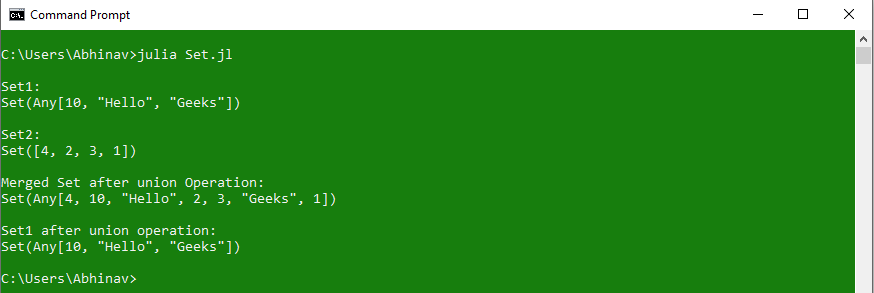Example 2: Performing union! operation

 `# Sets taken from previous example ` ` `  `# Performing Merge using union ! operation ` `println(``"\nMerged Set after union ! Operation:"``) ` `println(union !(Set1, Set2)) ` ` `  `# Existing set after union ! operation ` `println(``"\nSet1 after union ! operation:"``)  ` `println(Set1)  `

Output: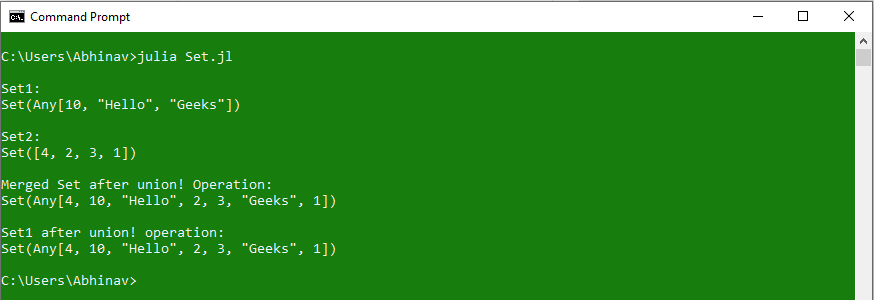As can be seen in the above code, `union!` operator after performing the merge operation updated the existing content of the Set1, but the `union` operator doesn’t make any changes to the existing sets and only performs the merge operation.

#### Intersection of Sets

Julia allows finding common elements of two sets with the use of `intersect` keyword. Intersection operation takes both the sets as an argument and gives the result as another set having common elements of both the sets. The actual values of both sets do not change unless the return value is stored in any of the two sets. There’s another variant of `intersect` function which is `intersect!`. Both the keywords perform similar operations, but the only difference is that the `intersect!` function will overwrite the existing set with the new intersected set.

Syntax:

```intersect(Set1, Set2)
or
intersect!(Set1, Set2)
```

Example 1: Performing intersect operation

 `# Julia program to demonstrate  ` `# Accessing of elements in a set  ` `   `  `# Creating a set  ` `Set1 ``=` `Set``([``"Hello"``, ``"Geeks"``, ``1``, ``2``, ``3``])  ` `println(``"\nSet1:"``)  ` `println(Set1)  ` ` `  `# Creating another set ` `Set2 ``=` `Set``([``1``, ``2``, ``3``, ``4``, ``"Geeks"``]) ` `println(``"\nSet2:"``) ` `println(Set2) ` ` `  `# Performing intersection using 'intersect' operation ` `println(``"\nSet after intersect Operation:"``) ` `println(intersect(Set1, Set2)) ` ` `  `# Existing set after intersect operation ` `println(``"\nSet1 after intersect operation:"``)  ` `println(Set1)  `

Output: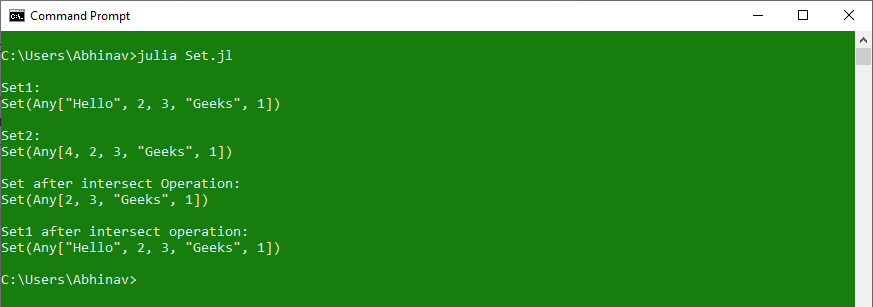Example 2: Performing intersect! operation

 `# Performing intersection using 'intersect !' operation ` `println(``"\nSet after intersect ! Operation:"``) ` `println(intersect !(Set1, Set2)) ` ` `  `# Existing set after intersect ! operation ` `println(``"\nSet1 after intersect ! operation:"``)  ` `println(Set1)  `

Output: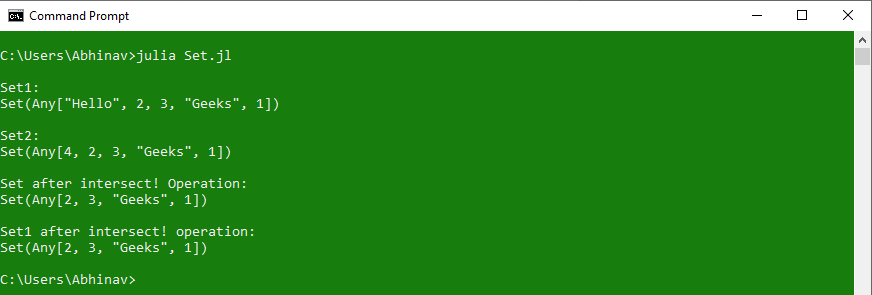As can be seen in the above code, `intersect!` operator after performing the intersection updated the existing content of the Set1, but the `intersect` operator doesn’t make any changes to the existing sets and only performs the intersection.

#### Difference between two Sets

Julia allows finding different elements between two sets with the use of `setdiff` keyword. This operator takes both the sets as an argument and gives the result as another set having different elements of both the sets. The actual values of both sets do not change unless the return value is stored in any of the two sets. There’s another variant of `setdiff` function which is `setdiff!`. Both the keywords perform similar operations, but the only difference is that the `setdiff!` function will overwrite the existing set with the new set.

Syntax:

```setdiff(Set1, Set2)
or
setdiff!(Set1, Set2)
```

Example 1: Performing setdiff operation

 `# Julia program to demonstrate  ` `# Accessing of elements in a set  ` `   `  `# Creating a set  ` `Set1 ``=` `Set``([``"Hello"``, ``"Geeks"``, ``1``, ``2``, ``3``])  ` `println(``"\nSet1:"``)  ` `println(Set1)  ` ` `  `# Creating another set ` `Set2 ``=` `Set``([``1``, ``2``, ``3``, ``4``, ``"Geeks"``]) ` `println(``"\nSet2:"``) ` `println(Set2) ` ` `  `# Finding Difference using 'setdiff' operation ` `println(``"\nSet after 'setdiff' Operation:"``) ` `println(setdiff(Set1, Set2)) ` ` `  `# Existing set after 'setdiff' operation ` `println(``"\nSet1 after 'setdiff' operation:"``)  ` `println(Set1)  `

Output: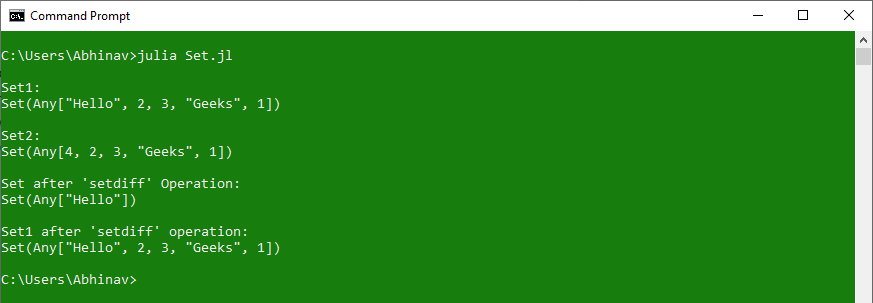Example 2: Performing setdiff! operation

 `# Finding Difference using 'setdiff !' operation ` `println(``"\nSet after 'setdiff !' Operation:"``) ` `println(setdiff !(Set1, Set2)) ` ` `  `# Existing set after 'setdiff !' operation ` `println(``"\nSet1 after 'setdiff !' operation:"``)  ` `println(Set1)  `

Output: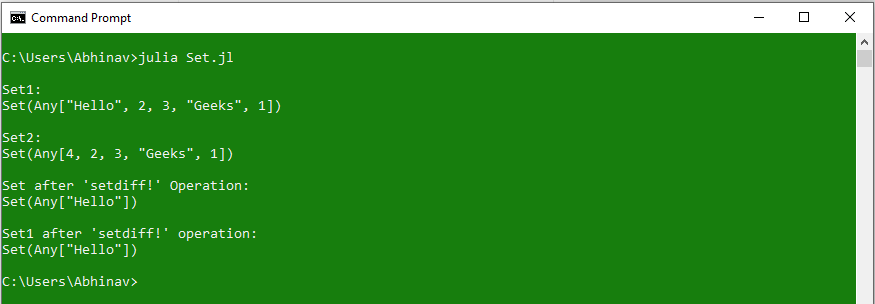As can be seen in the above code, `setdiff!` operator after performing the operation updated the existing content of the Set1, but the `setdiff` operator doesn’t make any changes to the existing sets and only gives the set of different elements.

#### Removing elements from a Set

Removing elements from a set is quite an easier task. Julia provides a pre-defined keyword `delete!` to remove a specific element from a set. Just like the addition process, removal of element is not possible from any specific index because of its unordered nature. The exact element is to be passed to the delete! function as an argument in order to remove it from the set.

Syntax:

```delete!(Set_name, value)
```

 `# Julia program to demonstrate  ` `# Accessing of elements in a set  ` `   `  `# Creating a set  ` `Set1 ``=` `Set``([``"Hello"``, ``"Geeks"``, ``1``, ``2``, ``3``])  ` `println(``"\nInitial set:"``)  ` `println(Set1)  ` ` `  `# Removing an element from the set ` `Set1 ``=` `delete !(Set1, ``"Hello"``) ` `println(``"\nSet after deleting one element:\n"``, Set1) ` ` `  `# Deleting whole set ` `for` `i ``in` `Set1 ` `    ``Set1 ``=` `delete !(Set1, i) ` `end ` ` `  `println(``"\nEmpty set after deleting all elements:\n"``, Set1) `

Output: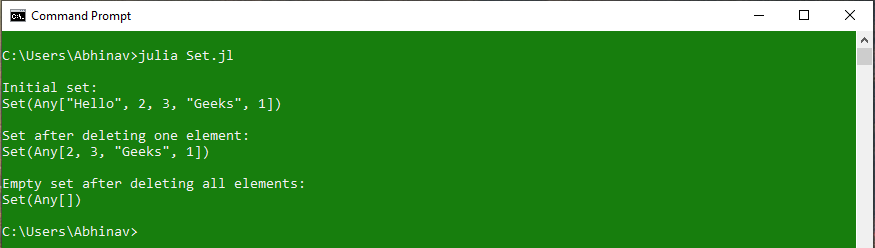#### Set Methods

Methods Description
intersect() Used to construct the intersection of the specified sets
intersect!() Used to intersect all passed in sets and overwrite s with the result
issetequal() Used to determine whether the two specified sets a and b have the same elements or not
setdiff() Used to returns the elements which are in first set but not in second set
setdiff!() Used to remove each element of set s which are also in the specified iterable
symdiff() Used to construct the symmetric difference of elements in the passed in sets
symdiff!() Used to construct the symmetric difference of the passed in sets, and overwrite the spcified set s with the result
union() Used to construct the union of the specified sets
union!() Used to construct the union of passed in sets and overwrite s with the result

My Personal Notes arrow_drop_upCheck out this Author's contributed articles.

If you like GeeksforGeeks and would like to contribute, you can also write an article using contribute.geeksforgeeks.org or mail your article to contribute@geeksforgeeks.org. See your article appearing on the GeeksforGeeks main page and help other Geeks.

Please Improve this article if you find anything incorrect by clicking on the "Improve Article" button below.

Article Tags :

Be the First to upvote.

Please write to us at contribute@geeksforgeeks.org to report any issue with the above content.